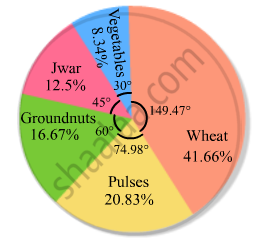Share

# Percentage of the Different Products of a Village in a Particular District Are Given Below. Draw a Pie-chart Representing this Information. - Mathematics

Course
ConceptCircle Graph Or Pie Chart - Drawing Pie Charts

#### Question

Percentage of the different products of a village in a particular district are given below. Draw a pie-chart representing this information.

 Items Wheat Pulses Jwar Grounnuts Vegetables Total % 125/3 125/6 25/2 50/3 25/3 100

#### Solution

We know:
Central angle of a component = (component value/sum of component values x 360ο)
Here, the total % of items = 100
Thus, the central angle for each component can be calculated as follows:

 Item In % Sector angle Wheat 125/3 41.66 41.66/100 x 360 = 149.97 Pulses 125/6 20.83 20.83/100 x 360 = 74.98 Jwar 25/2 12.5 12.5/100 x 360 = 45 Groundnuts 50/3 16.66 16.66/100 x 360 = 59.97 Vegetables 25/3 8.33 8.33/100 x 360 = 29.98

Now, the pie chat representing the given data can be constructed by following the steps below:
Step 1 : Draw circle of an appropriate radius.
Step 2 : Draw a vertical radius of the circle drawn in step 1.
Step 3 : Choose the largest central angle. Here the largest central angle is 149.97o. Draw a sector with the central angle 149.97o in such a way that one of its radii coincides with the radius drawn in step 2 and another radius is in its counter clockwise direction.
Step 4 : Construct other sectors representing other items in the clockwise sense in descending order of magnitudes of their central angles.
​Step 5 : Shade the sectors with different colours and label them, as shown as in the figure below.​​Is there an error in this question or solution?

#### APPEARS IN

RD Sharma Solution for Mathematics for Class 8 by R D Sharma (2019-2020 Session) (2017 to Current)
Chapter 25: Data Handling-III (Pictorial Representation of Data as Pie Charts or Circle Graphs)
Ex. 25.1 | Q: 7 | Page no. 12

#### Video TutorialsVIEW ALL 

Solution Percentage of the Different Products of a Village in a Particular District Are Given Below. Draw a Pie-chart Representing this Information. Concept: Circle Graph Or Pie Chart - Drawing Pie Charts.
S